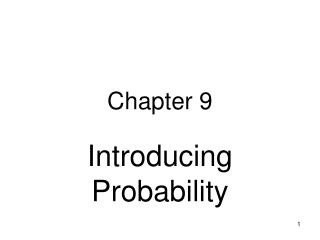# Chapter 9 - PowerPoint PPT PresentationDownload PresentationChapter 9

Chapter 9
Download Presentation## Chapter 9

- - - - - - - - - - - - - - - - - - - - - - - - - - - E N D - - - - - - - - - - - - - - - - - - - - - - - - - - -
##### Presentation Transcript

1. Chapter 9 Introducing Probability

2. From Exploration to Inferencep. 150 in text

3. The Idea of Probability Example: A random sample of n = 100 children has 8 individuals with asthma. What is the probability a child has asthma? • Probabilityhelps us deal with “chance” • Definition: the probability of an event is its expected proportion in an infinite series of repetitions ANS: We do not know. Although 8% is a reasonable “guesstimate,” the true probability is not known because our sample was not infinitely large

4. How Probability BehavesCoin Toss Example Chancebehavior is unpredictable in the short run, but is predictable in the long run. The proportion of heads approaches 0.5 with many, many tosses.

5. Probability Models • Probability models consist of these two parts: • Sample Space (S) = the set of all possible outcomes of a random process • Probabilities (Pr) for each possible outcome in the sample space Example of a probability model “Toss a fair coin once” S = {Heads, Tails} all possible outcomes Pr(heads) = 0.5 and Pr(tails) = 0.5probabilities for each outcome

6. 0 ≤ Pr(A) ≤ 1 Pr(S) = 1 Addition Rule for Disjoint Events Law of Complements 4 Basic Rules of Probability (Summary) Also on bottom of page 1 of Formula Sheet

7. Rule 1 (Range of Possible Probabilities) Let A ≡ event A Pr(A) ≡ probability of event A Rule 1 says “0 ≤ Pr(A) ≤ 1” Probabilities are always between 0 & 1 Pr(A) = 0 means A never occurs Pr(A) = 1 means A always occurs Pr(A) = .25 means A occurs 25% of the time Pr(A) = 1.25  Impossible! Must be something wrong Pr(A) = some negative number  Impossible! Must be something wrong

8. Rule 2 (Sample Space Rule) Let S ≡ the Sample Space Pr(S) = 1 All probabilities in the sample space must sum to 1 exactly. Example: “toss a fair coin” S = {heads or tails} Pr(heads) + Pr(tails) = 0.5 + 0.5 = 1.0

9. Rule 3 (Addition Rule, Disjoint Events) Events A and B are disjoint if they can never occur together. When events are disjoint: Pr(A or B) = Pr(A) + Pr(B) Age of mother at first birth Let A ≡ first birth at age < 20: Pr(A) = 25% Let B ≡ first birth at age 20 to 24: Pr(B) = 33% Let C ≡ age at first birth ≥25 Pr(C) = 42% Probability age at first birth ≥ 20 = Pr(B or C) = Pr(B) + Pr(C) = 33% + 42% = 75%

10. Rule 4 (Rule of Complements) Let Ā≡ A does NOT occur This is called the complement of event A Pr(Ā) = 1 – Pr(A) Example: If A ≡ “survived” then  Ā ≡ “did not survive” If Pr(A) = 0.9 then  Pr(Ā) = 1 – Pr(A) = 1 – 0.9 = 0.1

11. Probability Mass Functions pmfs Probability mass functions are made up of a list of separated outcomes. For discrete random variables. Example of a pmf: A couple wants three children. Let X ≡ the number of girls they will have Here is the pmf that suits this situation:

12. To assign probabilities for continuous random variables  we density curve Properties of a density curve Always on or above horizontal axis Has total area under curve (AUC) of exactly 1 AUC in any range = probability of a value in that range Probability Density Functions pdfs (“Density Curves”) Probability density functions form a continuum of possible outcomes. For continuous random variables.

13. Note The curve is always on or above horizontal axis and has AUC = height × base = 1 × 1 = 1 Probability = AUC in the range. Examples follow. Pr(X < .5) = height × base = 1 × .5 = .5 Pr(X > 0.8) = height × base = 1 × .2 = .2 Pr (X < .5 or X > 0.8) = .5 + .2 = .7 Example of a pdf This random spinner has this pdf density“curve”

14. pdf Density Curves • Density curves come in many shapes • Prior slide showed a “uniform” shape • Below are “Normal” and “skewed right” shapes • Measures of center apply to density curves • µ (expected value or “mean”) is the center balancing point • Median splits the AUC in half

15. From Histogram to Density Curve Histograms show distribution in chunks The smooth curve drawn over the histogram represents a Normal density curve for the distribution 8/14/2014 15

16. Area Under the Curve (AUC) Area in Bars = proportion in that range 30% of students had scores ≤ 6 30% of students had scores ≤ 6 Shaded area = 30% of total area of the histogram 30% 70% 8/14/2014 16

17. Area Under the Curve (AUC) Area Under Curve = proportion in that range! 30% of students had scores ≤ 6 30% of area under the curve (AUC) is shaded 30% 70% 8/14/2014 17

18. Summary of Selected Points • To date we have studied descriptive statistics. From here forward we study inferential statistics {2} • Probability is the study chance; chance is unpredictable in the short run but is predictable in the long run {3 - 4}; take the rules of probability to heart{5 - 10} • Discrete random variables are described with probability mass function • Continuous random variables are described with density curves with the area under the curve (AUC) corresponding to probabilities